# ICSE Class 8 Maths Selina Solutions Chapter 3 Squares And Square Roots

Chapter 3 of Class 8 Maths “Square and Square Roots” explains different methods of calculating the square roots of numbers. Before learning about square roots, it is necessary for the students to first know about squares. We can find out the square of any number by simply multiplying a number by itself. For eg: A square root of 9 is … 3 because when 3 is multiplied by itself we get 9. The perfect squares are the squares of the whole numbers such as 1, 4, 9, 16, etc. While finding the square roots, positive number has two square roots; a positive (called the principal square root) and a negative.

Students of Class 8 ICSE should practice problems from the Selina textbook to score well in the annual exam. ICSE Class 8 Maths Selina Solutions PDF help students to cross check their answers and analyse their mistakes and weak points. But, before solving the questions they should have a strong hold about the topic “Square and Square Roots”.

## Download ICSE Class 8 Maths Selina Solutions PDF for Chapter 3:-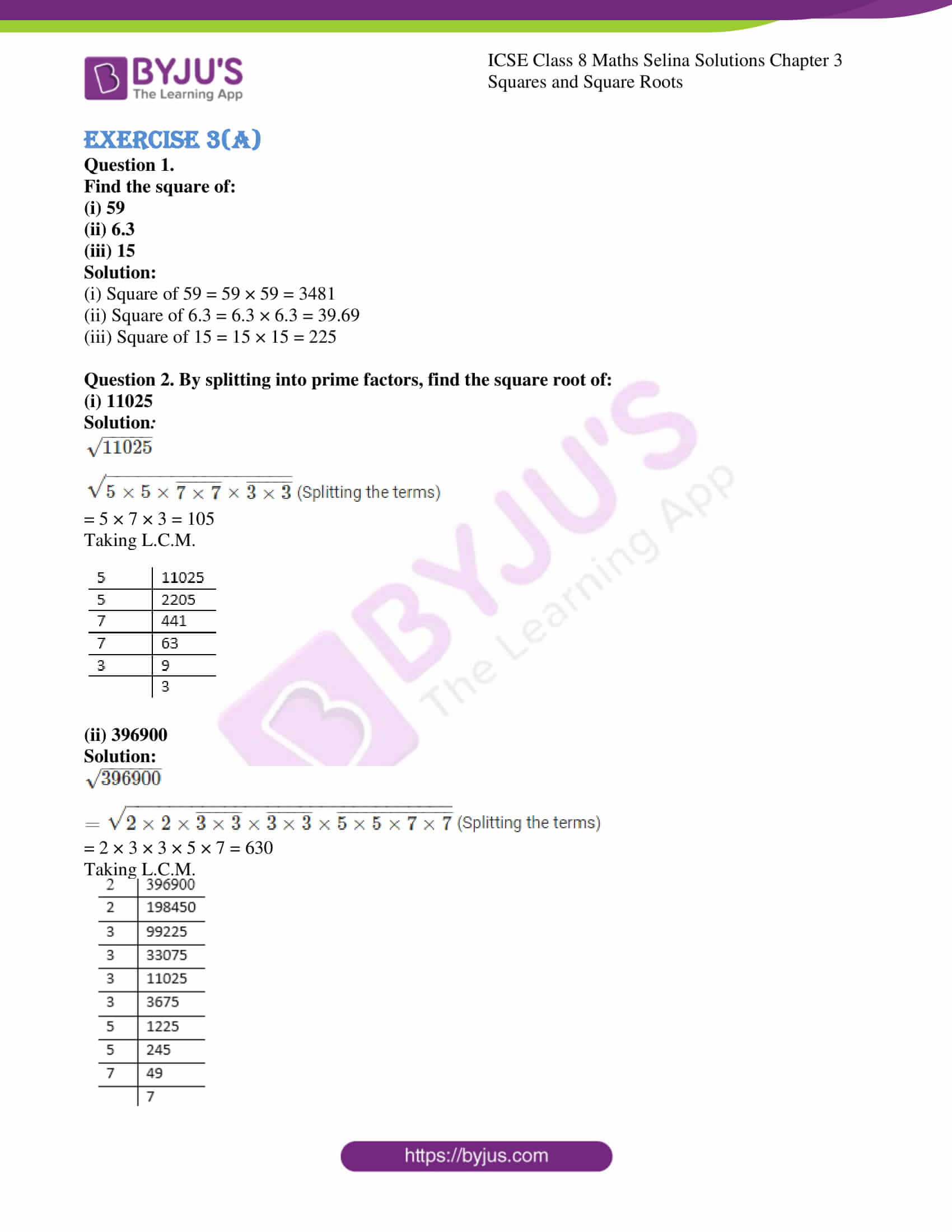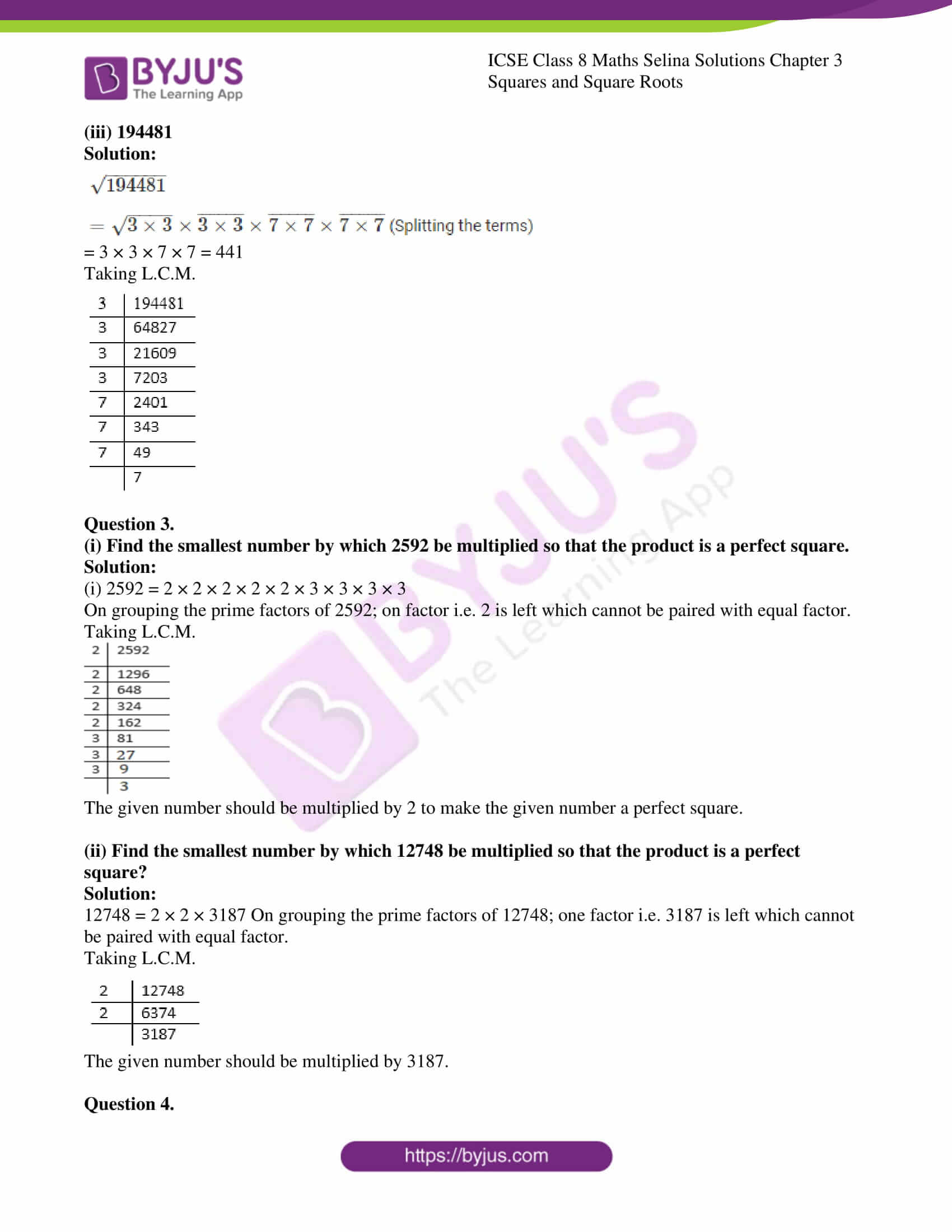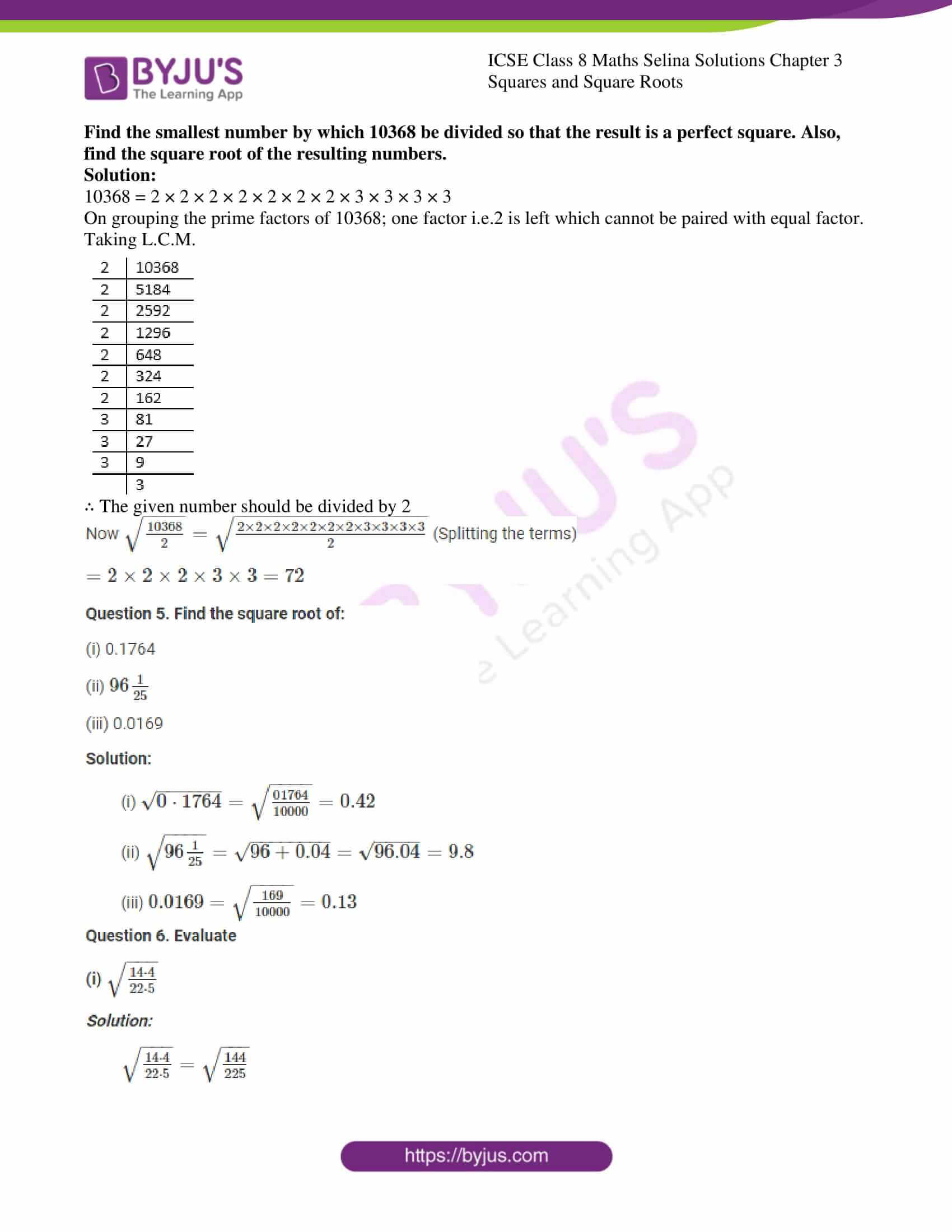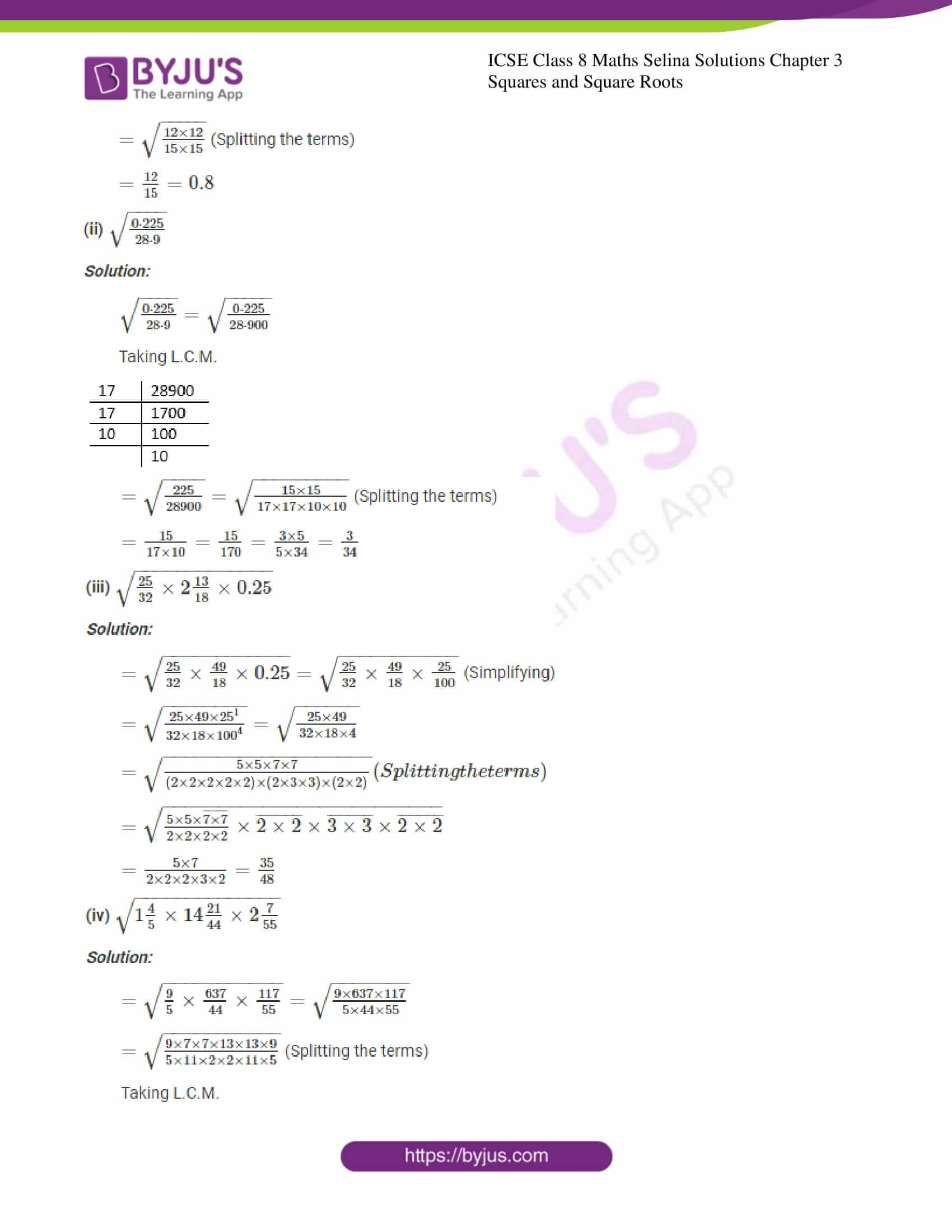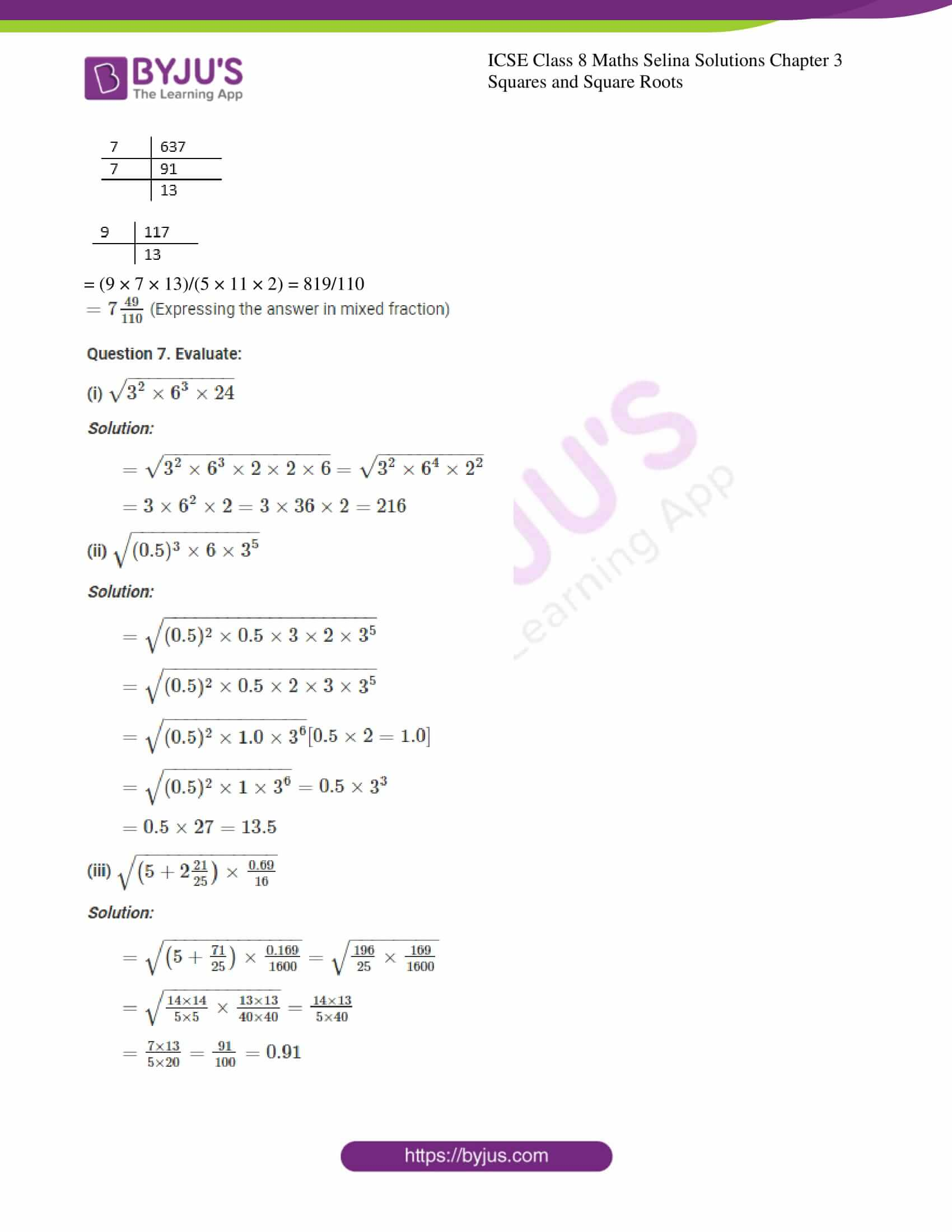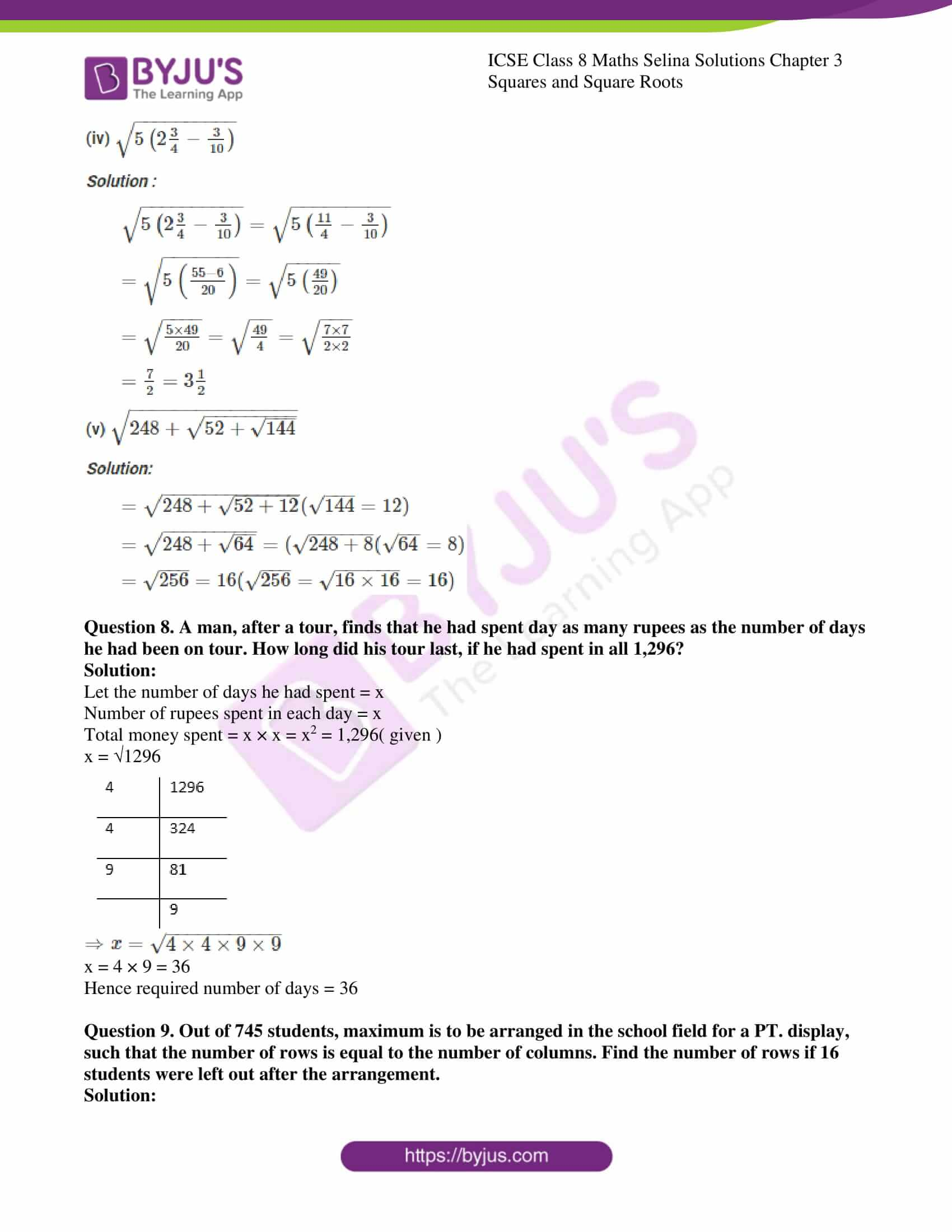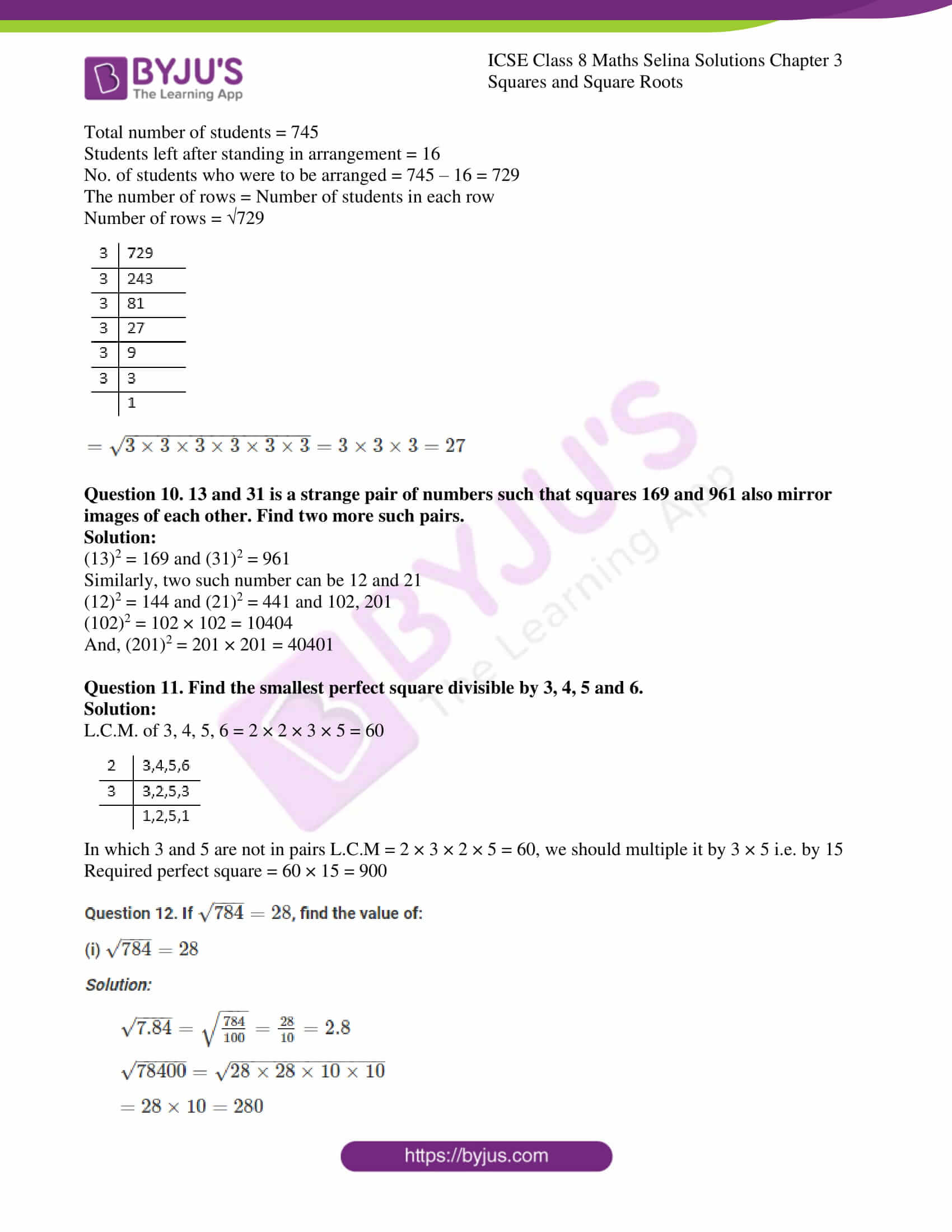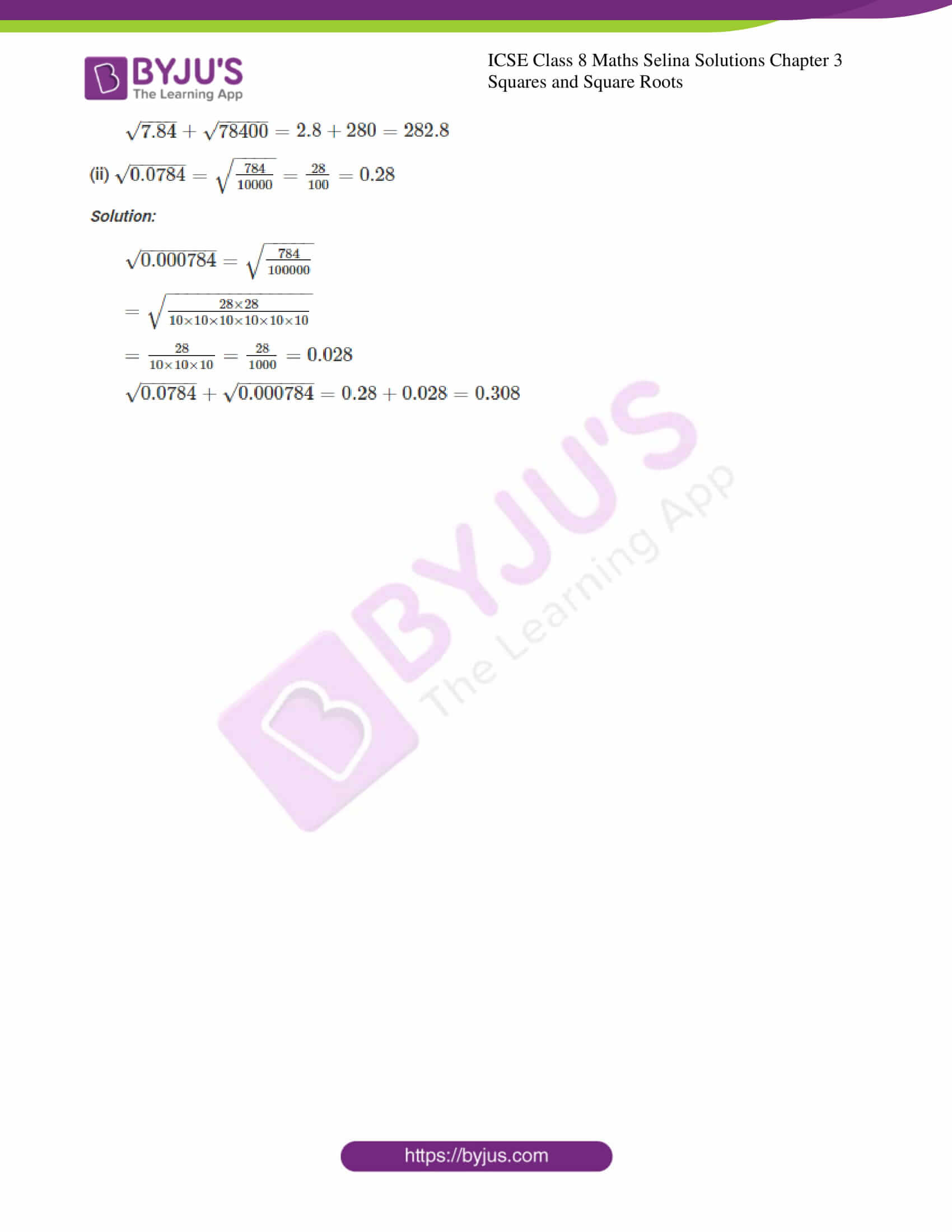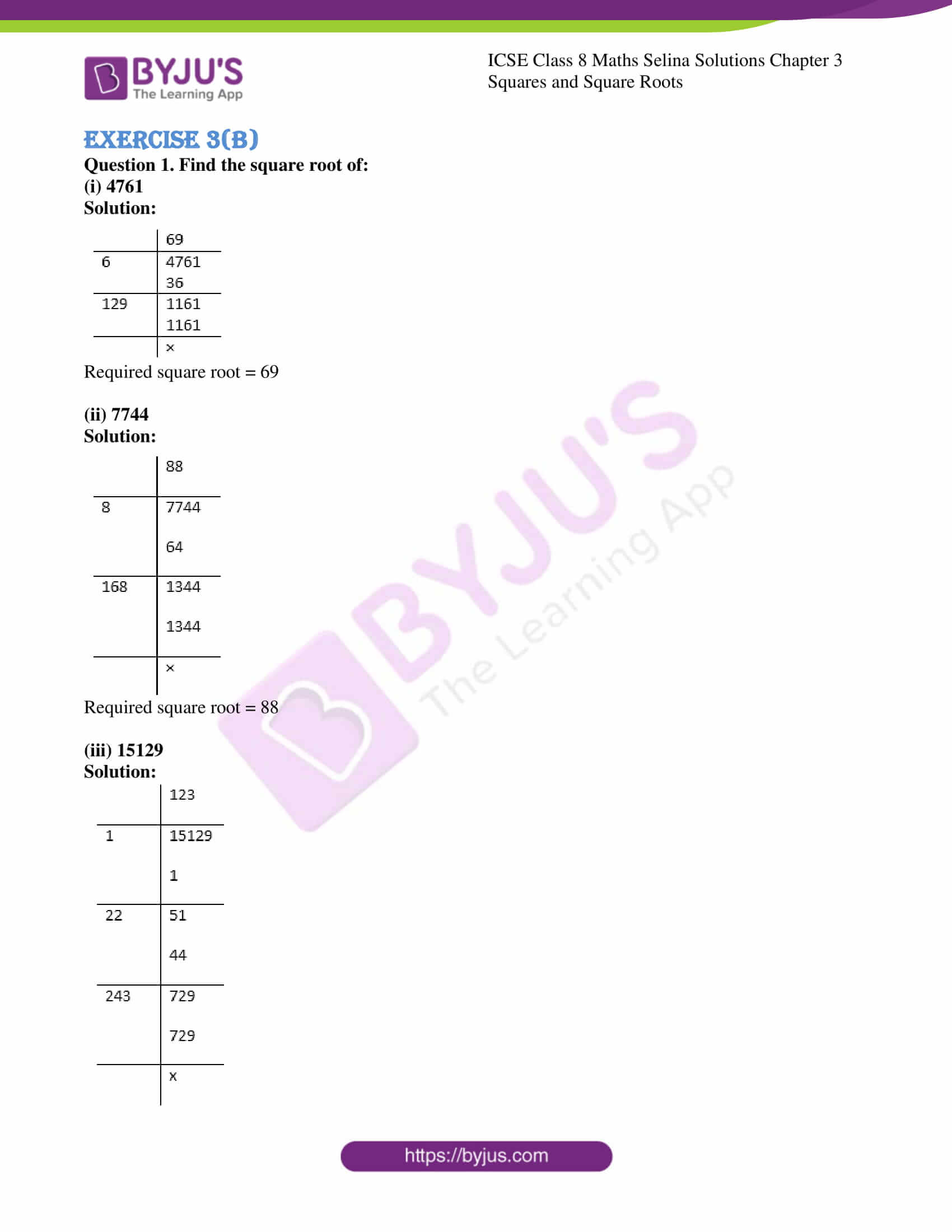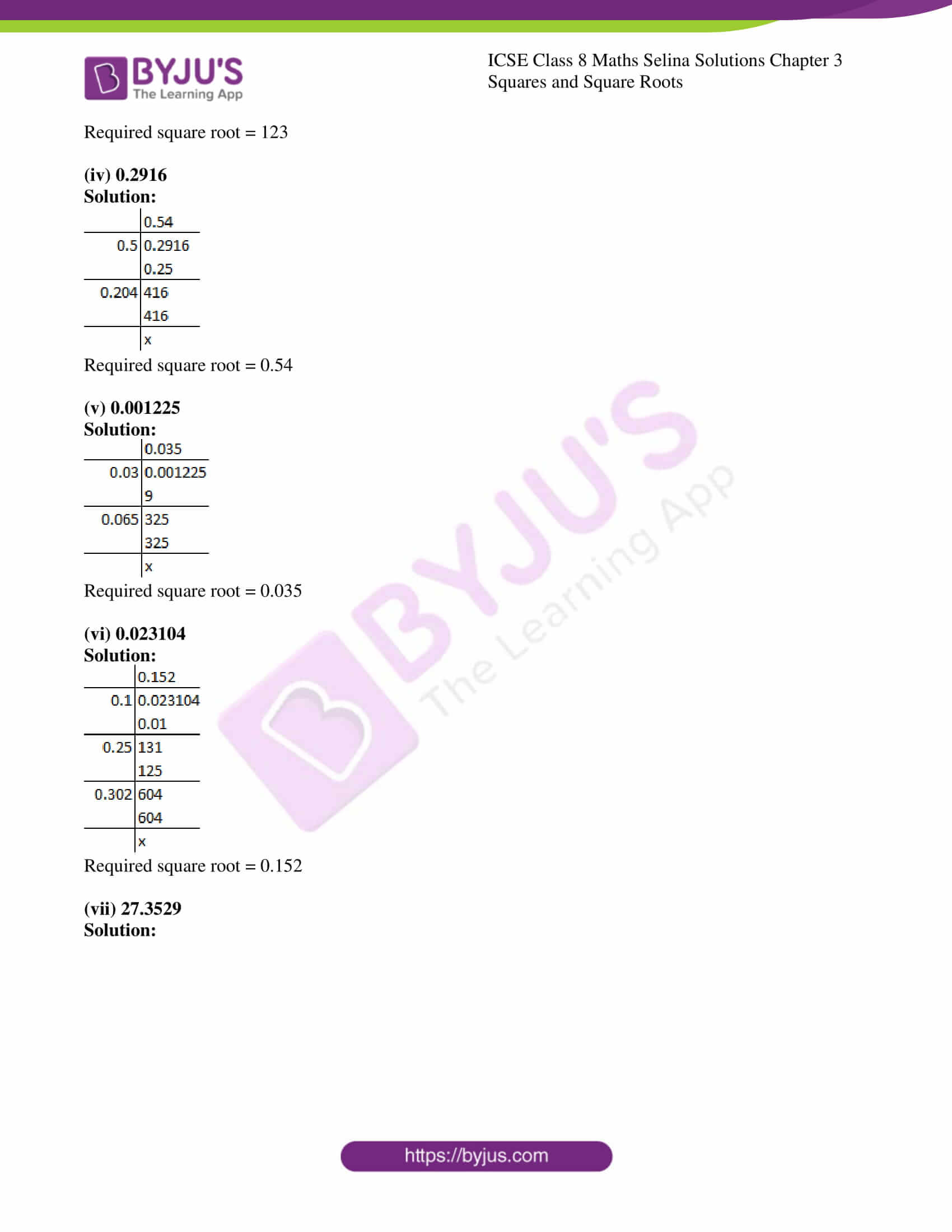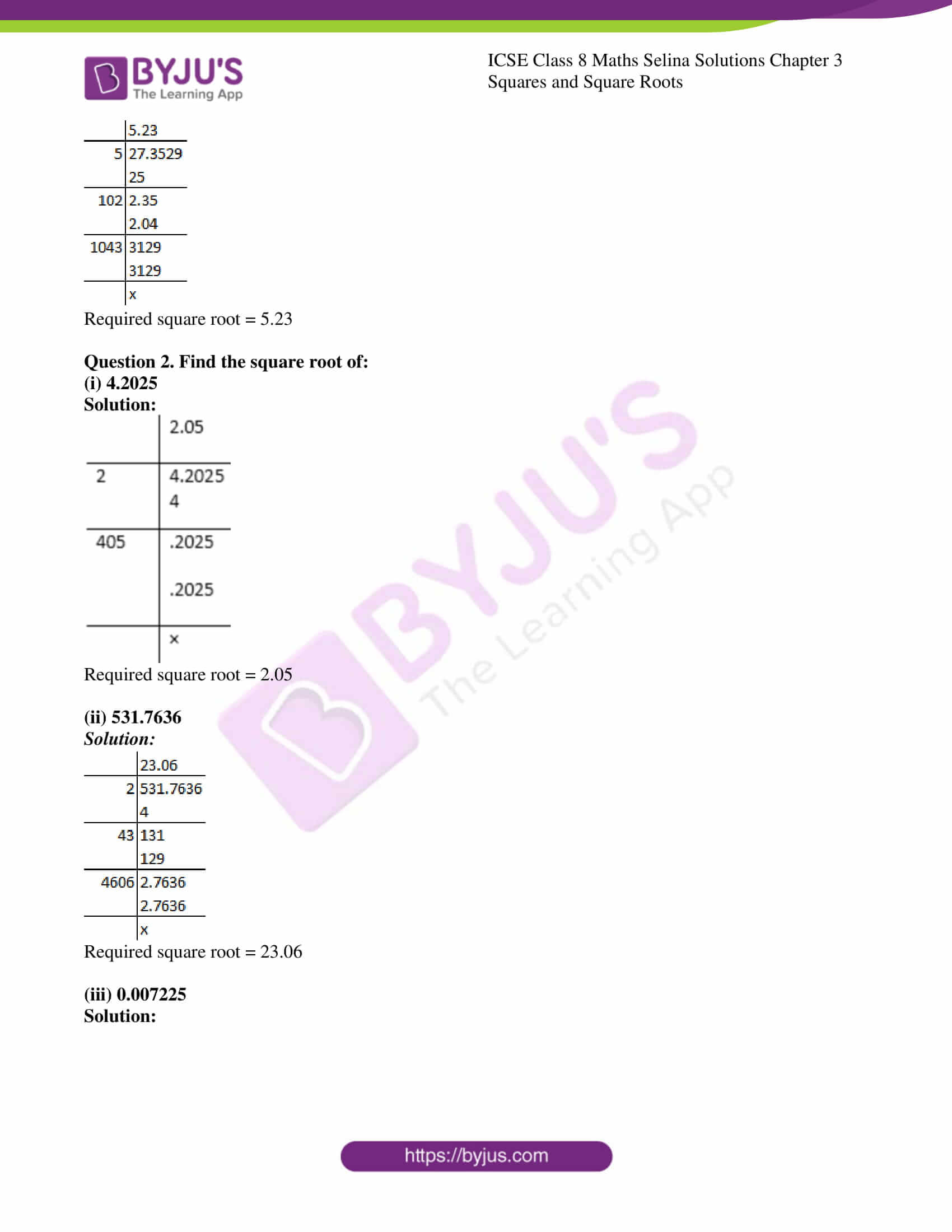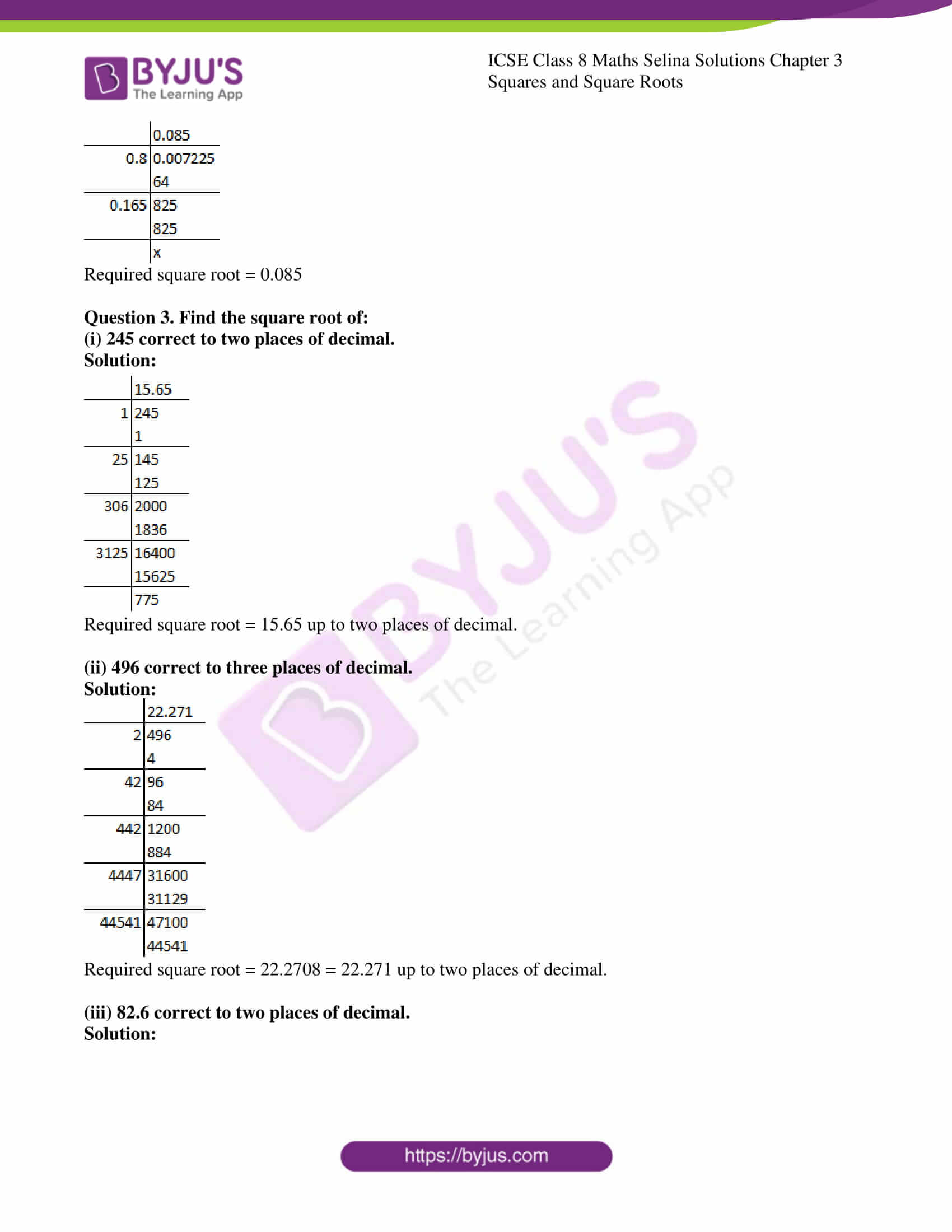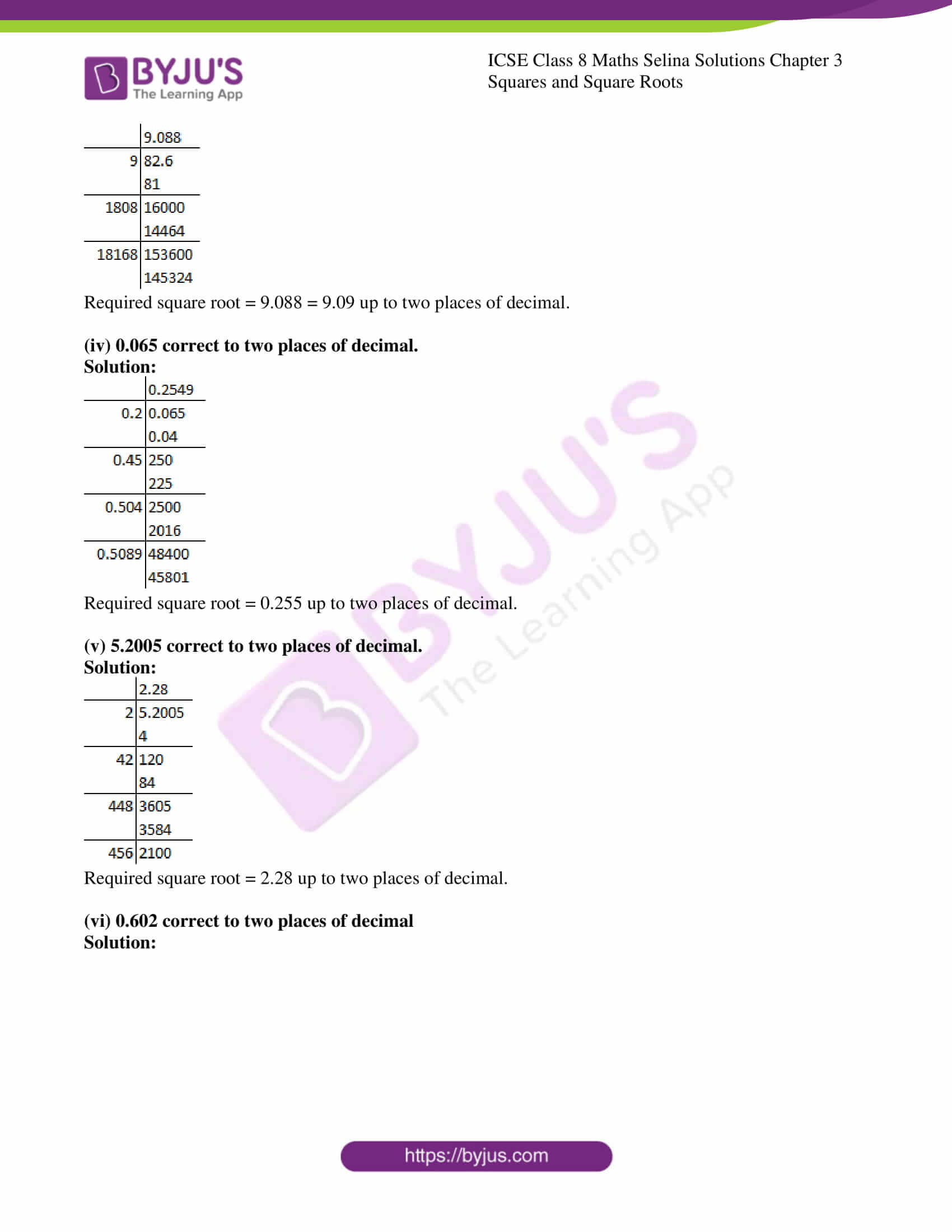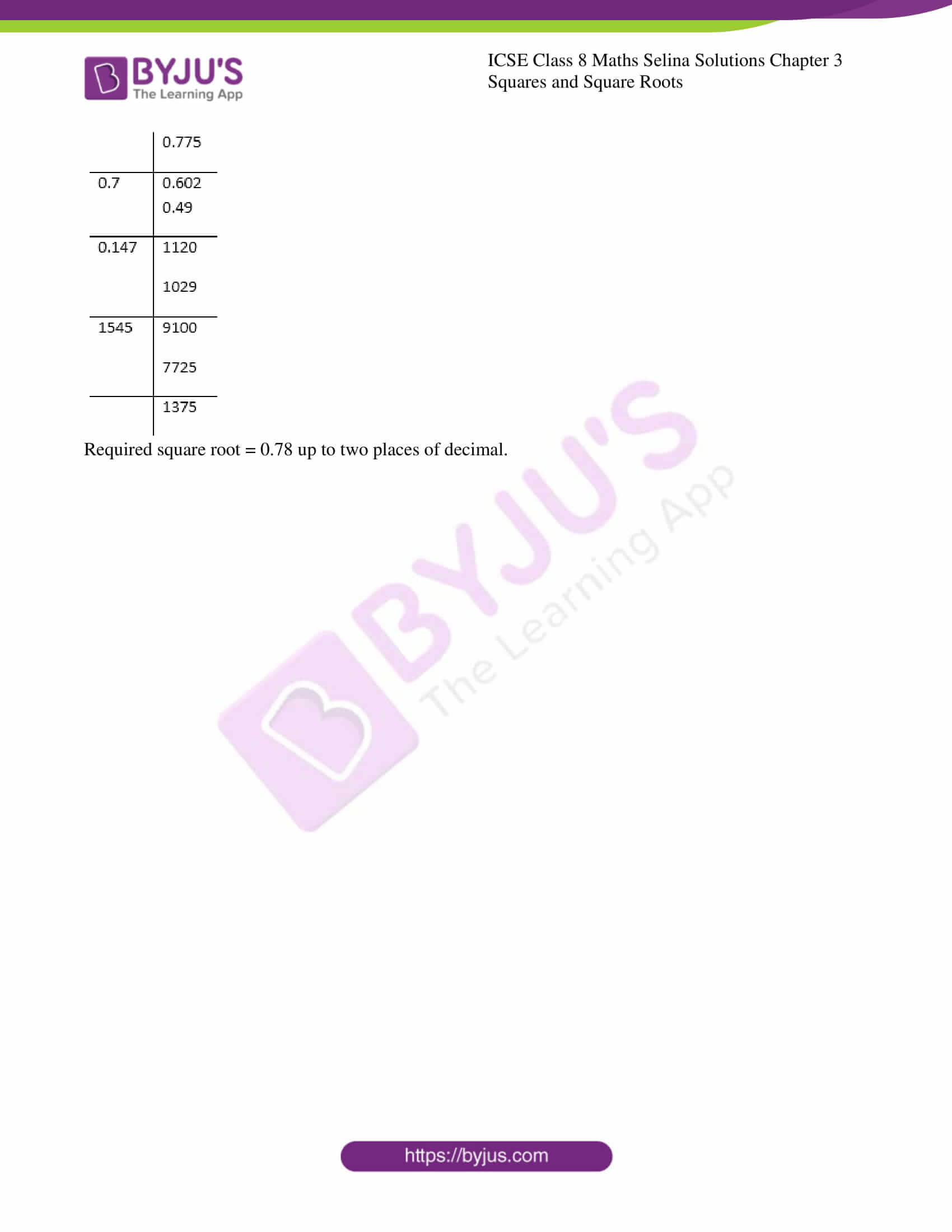### ICSE Class 8 Maths Selina Solutions Chapter 3 Squares And Square Roots – Exercise 3 (A)

Question 1.
Find the square of:
(i) 59

(ii) 6.3

(iii) 15

Solution:

(i) Square of

$$\begin{array}{l} 59=59 \times 59=3481\end{array}$$

(ii) Square of

$$\begin{array}{l} 6.3=6.3 \times 6.3=39.69 \end{array}$$

(iii) Square of

$$\begin{array}{l} 15=15 \times 15=225 \end{array}$$

Question 2. By splitting into prime factors, find the square root of:

(i) 11025

Solution:

$$\begin{array}{l} \sqrt{11025}\end{array}$$

$$\begin{array}{l} \sqrt{5 \times 5 \times \overline{7 \times 7} \times \overline{3 \times 3}}\end{array}$$
(Splitting the terms)

$$\begin{array}{l} =5 \times 7 \times 3=105\end{array}$$

Taking L.C.M.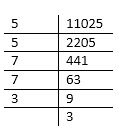(ii) 396900

Solution:

$$\begin{array}{l} \sqrt{396900}\end{array}$$

$$\begin{array}{l} =\sqrt{2 \times 2 \times \overline{3 \times 3} \times \overline{3 \times 3} \times \overline{5 \times 5 \times 7 \times 7}}\end{array}$$
(Splitting the terms)

$$\begin{array}{l} =2 \times 3 \times 3 \times 5 \times 7=630\end{array}$$

Taking L.C.M.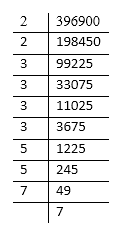(iii) 194481

Solution:

$$\begin{array}{l} \sqrt{194481}\end{array}$$

$$\begin{array}{l} =\sqrt{3 \times 3} \times \overline{3 \times 3} \times \overline{7 \times 7} \times \overline{7 \times 7}\end{array}$$
(Splitting the terms)

$$\begin{array}{l} =3 \times 3 \times 7 \times 7=441\end{array}$$

Taking L.C.M.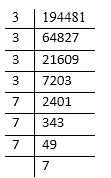Question 3.
(i) Find the smallest number by which 2592 be multiplied so that the product is a perfect square.

Solution:

(i)

$$\begin{array}{l} 2592=2 \times 2 \times 2 \times 2 \times 2 \times 3 \times 3 \times 3 \times 3\end{array}$$

On grouping the prime factors of 2592; on factor i.e. 2 is left which cannot be paired with equal factor.

Taking L.C.M.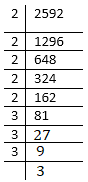The given number should be multiplied by 2 to make the given number a perfect square.

(ii) Find the smallest number by which 12748 be multiplied so that the product is a perfect square?

Solution:

$$\begin{array}{l} 12748=2 \times 2 \times 3187\end{array}$$
On grouping the prime factors of 12748; one factor i.e. 3187 is left which cannot be paired with equal factor.
Taking L.C.M.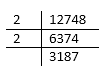The given number should be multiplied by 3187.

Question 4.

Find the smallest number by which 10368 be divided so that the result is a perfect square. Also, find the square root of the resulting numbers.

Solution:

$$\begin{array}{l} 10368=2 \times 2 \times 2 \times 2 \times 2 \times 2 \times 2 \times 3 \times 3 \times 3 \times 3\end{array}$$

On grouping the prime factors of 10368; one factor i.e.2 is left which cannot be paired with equal factor.

Taking L.C.M.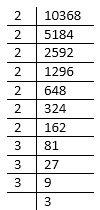∴ The given number should be divided by 2

Now

$$\begin{array}{l} \sqrt{\frac{10368}{2}}=\sqrt{\frac{2 \times 2 \times 2 \times 2 \times 2 \times 2 \times 2 \times 3 \times 3 \times 3 \times 3}{2}} \end{array}$$
(Splitting the terms)

$$\begin{array}{l} =2 \times 2 \times 2 \times 3 \times 3=72\end{array}$$

Question 5. Find the square root of:

(i) 0.1764

(ii)

$$\begin{array}{l} 96 \frac{1}{25}\end{array}$$

(iii) 0.0169

Solution:

(i)

$$\begin{array}{l} \sqrt{0 \cdot 1764}=\sqrt{\frac{01764}{10000}}=0.42\end{array}$$

(ii)

$$\begin{array}{l} \sqrt{96 \frac{1}{25}}=\sqrt{96+0.04}=\sqrt{96.04}=9.8\end{array}$$

(iii)

$$\begin{array}{l} 0.0169=\sqrt{\frac{169}{10000}}=0.13\end{array}$$

Question 6. Evaluate

(i)

$$\begin{array}{l} \sqrt{\frac{14 \cdot 4}{22 \cdot 5}} \end{array}$$

Solution:

$$\begin{array}{l} \sqrt{\frac{14 \cdot 4}{22 \cdot 5}}=\sqrt{\frac{144}{225}}\end{array}$$

$$\begin{array}{l} =\sqrt{\frac{12 \times 12}{15 \times 15}}\end{array}$$
(Splitting the terms)

$$\begin{array}{l} =\frac{12}{15}=0.8\end{array}$$

(ii)

$$\begin{array}{l} \sqrt{\frac{0 \cdot 225}{28 \cdot 9}} \end{array}$$

Solution:

$$\begin{array}{l} \sqrt{\frac{0 \cdot 225}{28 \cdot 9}}=\sqrt{\frac{0 \cdot 225}{28 \cdot 900}}\end{array}$$

Taking L.C.M.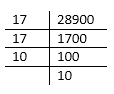$$\begin{array}{l} =\sqrt{\frac{225}{28900}}=\sqrt{\frac{15 \times 15}{17 \times 17 \times 10 \times 10}} \end{array}$$
(Splitting the terms)

$$\begin{array}{l} =\frac{15}{17 \times 10}=\frac{15}{170}=\frac{3 \times 5}{5 \times 34}=\frac{3}{34}\end{array}$$

(iii)

$$\begin{array}{l} \sqrt{\frac{25}{32} \times 2 \frac{13}{18} \times 0.25}\end{array}$$

Solution:

$$\begin{array}{l} =\sqrt{\frac{25}{32} \times \frac{49}{18} \times 0.25}=\sqrt{\frac{25}{32} \times \frac{49}{18} \times \frac{25}{100}}\end{array}$$
(Simplifying)

$$\begin{array}{l} =\sqrt{\frac{25 \times 49 \times 25^{1}}{32 \times 18 \times 100^{4}}}=\sqrt{\frac{25 \times 49}{32 \times 18 \times 4}}\end{array}$$

$$\begin{array}{l} =\sqrt{\frac{5 \times 5 \times 7 \times 7}{(2 \times 2 \times 2 \times 2 \times 2) \times(2 \times 3 \times 3) \times(2 \times 2)}} (Splitting the terms) \end{array}$$

$$\begin{array}{l} =\sqrt{\frac{5 \times 5 \times \overline{7 \times 7}}{2 \times 2 \times 2 \times 2} \times \overline{2 \times 2} \times \overline{3 \times 3} \times \overline{2 \times 2}}\end{array}$$

$$\begin{array}{l} =\frac{5 \times 7}{2 \times 2 \times 2 \times 3 \times 2}=\frac{35}{48}\end{array}$$

(iv)

$$\begin{array}{l} \sqrt{1 \frac{4}{5} \times 14 \frac{21}{44} \times 2 \frac{7}{55}}\end{array}$$

Solution:

$$\begin{array}{l} =\sqrt{\frac{9}{5} \times \frac{637}{44} \times \frac{117}{55}}=\sqrt{\frac{9 \times 637 \times 117}{5 \times 44 \times 55}}\end{array}$$

$$\begin{array}{l} =\sqrt{\frac{9 \times 7 \times 7 \times 13 \times 13 \times 9}{5 \times 11 \times 2 \times 2 \times 11 \times 5}}\end{array}$$
(Splitting the terms)

Taking L.C.M.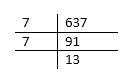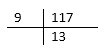$$\begin{array}{l} =\frac{9 \times 7 \times 13}{5 \times 11 \times 2}=\frac{819}{110}=7 \frac{49}{110} \end{array}$$
(Expressing the answer in mixed fraction)

Question 7. Evaluate:

(i)

$$\begin{array}{l} \sqrt{3^{2} \times 6^{3} \times 24}\end{array}$$

Solution:

$$\begin{array}{l} =\sqrt{3^{2} \times 6^{3} \times 2 \times 2 \times 6}=\sqrt{3^{2} \times 6^{4} \times 2^{2}}\end{array}$$

$$\begin{array}{l} =3 \times 6^{2} \times 2=3 \times 36 \times 2=216\end{array}$$

(ii)

$$\begin{array}{l} \sqrt{(0.5)^{3} \times 6 \times 3^{5}} \end{array}$$

Solution:

$$\begin{array}{l} =\sqrt{(0.5)^{2} \times 0.5 \times 3 \times 2 \times 3^{5}}\end{array}$$

$$\begin{array}{l} =\sqrt{(0.5)^{2} \times 0.5 \times 2 \times 3 \times 3^{5}}\end{array}$$

$$\begin{array}{l} =\sqrt{(0.5)^{2} \times 1.0 \times 3^{6}}[0.5 \times 2=1.0] \end{array}$$

$$\begin{array}{l} =\sqrt{(0.5)^{2} \times 1 \times 3^{6}}=0.5 \times 3^{3}\end{array}$$

$$\begin{array}{l} =0.5 \times 27=13.5\end{array}$$

(iii)

$$\begin{array}{l} \sqrt{\left(5+2 \frac{21}{25}\right) \times \frac{0.69}{16}} \end{array}$$

Solution:

$$\begin{array}{l} =\sqrt{\left(5+\frac{71}{25}\right) \times \frac{0.169}{1600}}=\sqrt{\frac{196}{25} \times \frac{169}{1600}}\end{array}$$

$$\begin{array}{l} =\sqrt{\frac{14 \times 14}{5 \times 5} \times \frac{13 \times 13}{40 \times 40}}=\frac{14 \times 13}{5 \times 40}\end{array}$$

$$\begin{array}{l} =\frac{7 \times 13}{5 \times 20}=\frac{91}{100}=0.91\end{array}$$

(iv)

$$\begin{array}{l} \sqrt{5\left(2 \frac{3}{4}-\frac{3}{10}\right)} \end{array}$$

Solution :

$$\begin{array}{l} \sqrt{5\left(2 \frac{3}{4}-\frac{3}{10}\right)}=\sqrt{5\left(\frac{11}{4}-\frac{3}{10}\right)} \end{array}$$

$$\begin{array}{l} =\sqrt{5\left(\frac{55-6}{20}\right)}=\sqrt{5\left(\frac{49}{20}\right)} \end{array}$$

$$\begin{array}{l} =\sqrt{\frac{5 \times 49}{20}}=\sqrt{\frac{49}{4}}=\sqrt{\frac{7 \times 7}{2 \times 2}}\end{array}$$

$$\begin{array}{l} =\frac{7}{2}=3 \frac{1}{2}\end{array}$$

(v)

$$\begin{array}{l} \sqrt{248+\sqrt{52+\sqrt{144}}}\end{array}$$

Solution:

$$\begin{array}{l} =\sqrt{248+\sqrt{52+12}} (\sqrt{144}=12) \end{array}$$

$$\begin{array}{l} =\sqrt{248+\sqrt{64}}=(\sqrt{248+8} ( \sqrt{64}=8) \end{array}$$

$$\begin{array}{l} =\sqrt{256}=16 (\sqrt{256}=\sqrt{16 \times 16}=16) \end{array}$$

Question 8. A man, after a tour, finds that he had spent day as many rupees as the number of days he had been on tour. How long did his tour last, if he had spent in all 1,296?

Solution:

Let the number of days he had spent =x
Number of rupees spent in each day =x
Total money spent

$$\begin{array}{l} =x \times x=x^{2}=1,296(\text { given })\end{array}$$

$$\begin{array}{l} x=\sqrt{1296}\end{array}$$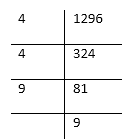$$\begin{array}{l} \Rightarrow x=\sqrt{4 \times 4 \times 9 \times 9}\end{array}$$

$$\begin{array}{l} x=4 \times 9=36\end{array}$$

Hence required number of days =36

Question 9. Out of 745 students, maximum is to be arranged in the school field for a PT. display, such that the number of rows is equal to the number of columns. Find the number of rows if 16 students were left out after the arrangement.

Solution:

Total number of students =745
Students left after standing in arrangement =16
No. of students who were to be arranged = 745-16=729
The number of rows = No. of students in each row
No. of rows

$$\begin{array}{l} =\sqrt{729}\end{array}$$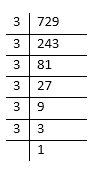$$\begin{array}{l} =\sqrt{3 \times 3 \times 3 \times 3 \times 3 \times 3}=3 \times 3 \times 3=27\end{array}$$

Question 10. 13 and 31 is a strange pair of numbers such that squares 169 and 961 also mirror images of each other. Find two more such pairs.

Solution:

$$\begin{array}{l} (13)^{2}=169 \text { and }(31)^{2}=961\end{array}$$

Similarly, two such number can be 12 and 21

$$\begin{array}{l} (12)^{2}=144 \text { and }(21)^{2}=441 and 102,201\end{array}$$

$$\begin{array}{l} (102)^{2}=102 \times 102=10404\end{array}$$

And,

$$\begin{array}{l} (201)^{2}=201 \times 201=40401\end{array}$$

Question 11. Find the smallest perfect square divisible by 3, 4, 5 and 6.

Solution:

L.C.M. of 3,4,5,6

$$\begin{array}{l} =2 \times 2 \times 3 \times 5=60\end{array}$$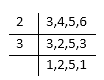In which 3 and 5 are not in pairs L•C.M
$$\begin{array}{l} =2 \times 3 \times 2 \times 5=60\end{array}$$
We should multiple it by 3×5 i.e. by 15
Required perfect square
$$\begin{array}{l} =60 \times 15=900\end{array}$$

Question 12. If

$$\begin{array}{l} \sqrt{784}=28\end{array}$$
, find the value of:

(i)

$$\begin{array}{l} \sqrt{784}=28\end{array}$$

Solution:

$$\begin{array}{l} \sqrt{7.84}=\sqrt{\frac{784}{100}}=\frac{28}{10}=2.8\end{array}$$

$$\begin{array}{l} \sqrt{78400}=\sqrt{28 \times 28 \times 10 \times 10}\end{array}$$

$$\begin{array}{l} =28 \times 10=280\end{array}$$

$$\begin{array}{l} \sqrt{7.84}+\sqrt{78400}=2.8+280=282.8\end{array}$$

(ii)

$$\begin{array}{l} \sqrt{0.0784}=\sqrt{\frac{784}{10000}}=\frac{28}{100}=0.28 \end{array}$$

Solution:

$$\begin{array}{l} \sqrt{0.000784}=\sqrt{\frac{784}{100000}}\end{array}$$

$$\begin{array}{l} =\sqrt{\frac{28 \times 28}{10 \times 10 \times 10 \times 10 \times 10 \times 10}}\end{array}$$

$$\begin{array}{l} =\frac{28}{10 \times 10 \times 10}=\frac{28}{1000}=0.028\end{array}$$

$$\begin{array}{l} \sqrt{0.0784}+\sqrt{0.000784}=0.28+0.028=0.308\end{array}$$

### ICSE Class 8 Maths Selina Solutions Chapter 3 Squares And Square Roots – Exercise 3 (B)

Question 1. Find the square root of:
(i) 4761

Solution: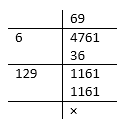Required square root =69

(ii) 7744

Solution: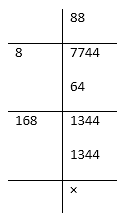Required square root =88

(iii) 15129

Solution: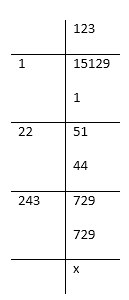Required square root =123

(iv) 0.2916

Solution: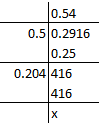Required square root =0.54

(v) 0.001225

Solution: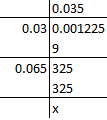Required square root =0.035

(vi) 0.023104

Solution: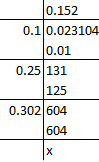Required square root =0.152

(vii) 27.3529

Solution: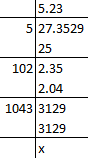Required square root =5.23

Question 2. Find the square root of:

(i) 4.2025

Solution: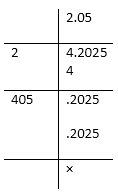Required square root =2.05

(ii) 531.7636

Solution: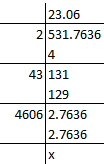Required square root =23.06

(iii) 0.007225

Solution: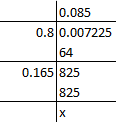Required square root =0.085

Question 3. Find the square root of:

(i) 245 correct to two places of decimal.

Solution: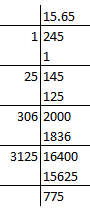Required square root =15.65 up to two places of decimal.

(ii) 496 correct to three places of decimal.

Solution: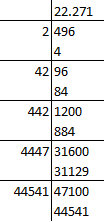Required square root = 22.2708=22.271 up to two places of decimal.

(iii) 82.6 correct to two places of decimal.

Solution: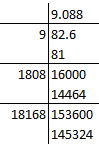Required square root =9.088=9.09 up to two places of decimal.

(iv) 0.065 correct to two places of decimal.

Solution: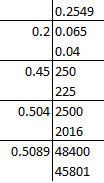Required square root =.255 up to two places of decimal.

(v) 5.2005 correct to two places of decimal.

Solution: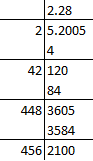Required square root =2.28 up to two places of decimal.

(vi) 0.602 correct to two places of decimal

Solution: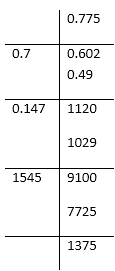Required square root =0.78 up to two places of decimal.